# Pseudo Phase Locked Loop with Arduino and X9C104 pot

How to use the Arduino with a X9C104 pot to implement a pseudo Phase Locked Loop square wave generator

IntermediateFull instructions provided2 hours226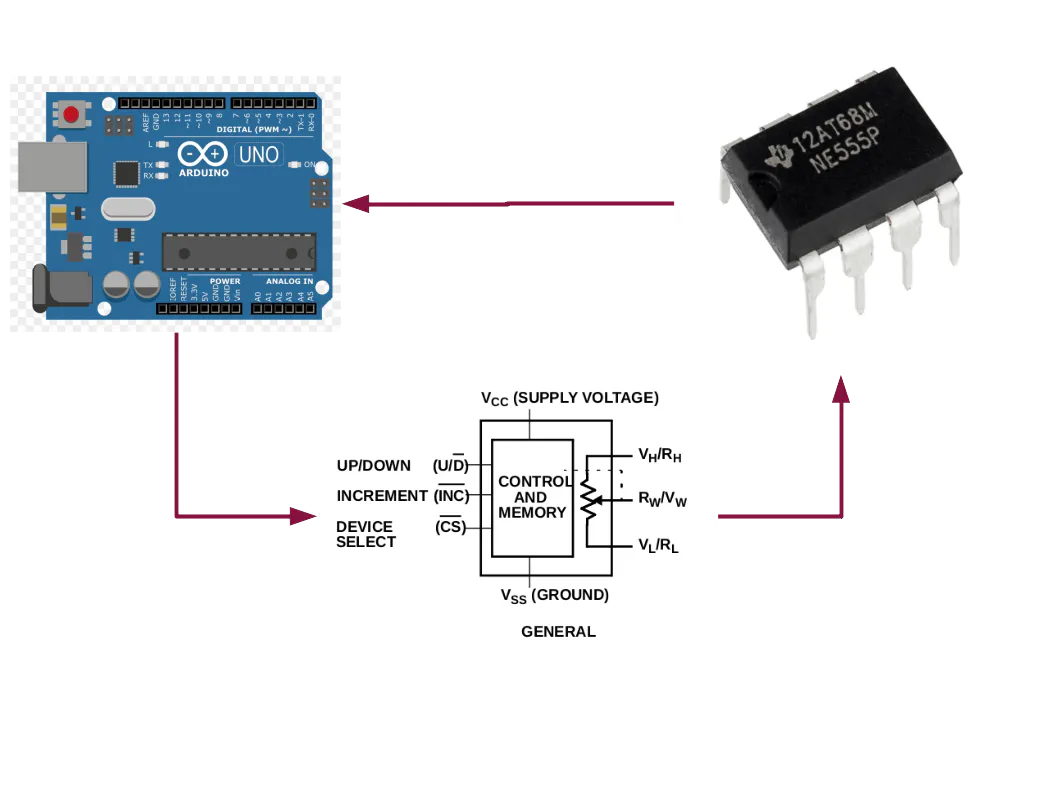## Things used in this project

### Hardware componentsArduino UNO
×1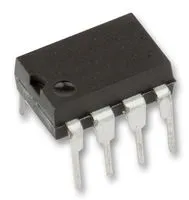555 Timers
×1Resistor 1k ohm
×1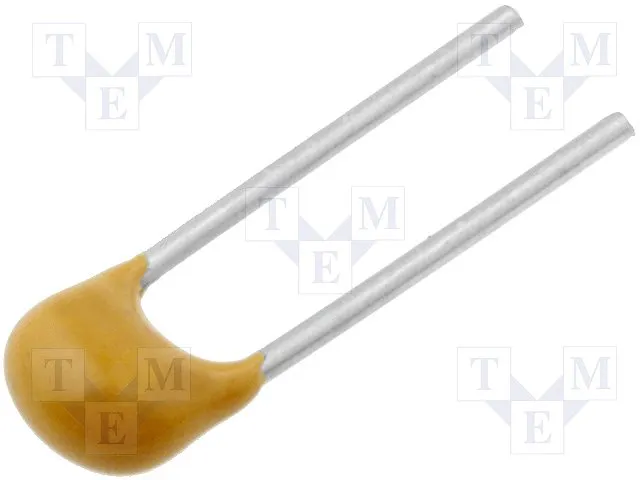Capacitor 100 nF
×1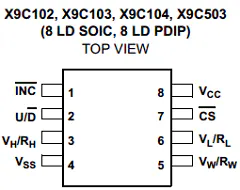Renesas X9C104
×1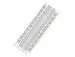×1

### Software apps and online servicesArduino IDE

## Schematics

### Schematic Diagram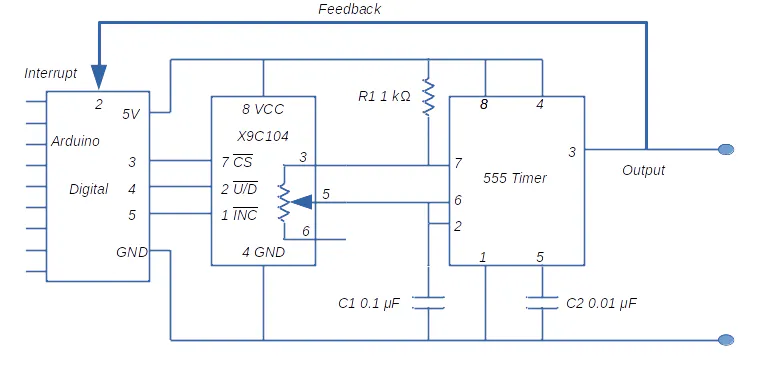## Code

### Arduino X9C104 in Feedback Loop

Arduino
Final code to fine tune circuit to user input frequency
```/*

This program uses a X9C104 potentiometer to control the frequency of oscillation
of a 555 timer circuit. The resistance value of the X9C104 is set by the Arduino
program. As frequency is the required output, a value (in Hz) is input by
the user via the serial monitor and then uses a feedback mechanism to set the
required output.

The feedback mechanism uses the interrrupt capability of the Arduino to measure
the 555 timer frequency. This frequency is then compared to the desired value
and the difference drives adjustments to the X9C104 potentiometer resistance.
This process tunes the 555 timer circuit such that the output frequency equals
the original input (within the limits of the potentiomenter step).

February 2022

*/
// Library which allows Arduino to control X9CXXX potentiometers
#include <FastX9CXXX.h>

#define X9_CS_PIN 3  //X9CXXX CS connected to digital pin 3 of Arduino
#define X9_UD_PIN 4  //X9CXXX UD connected to digital pin 4 of Arduino
#define X9_INC_PIN 5  //X9CXXX INC connected to digital pin 5 of Arduino

// Instantiate object for X9C104 control
FastX9C104 Potentiometer(X9_CS_PIN, X9_UD_PIN, X9_INC_PIN);

const byte interruptPin = 2;
const float cFactor = 7246377; //factor calculted from circuit component values
volatile float trig_time = 0;
volatile float last_trig_time = 0;
volatile bool trig_flag = false;
float trigArray ;
float interruptAvg;
float inputFrequency;
float actualFrequency;
float initialResistance;
float deltaResistance;
float finalResistance;
int potentiometerStep;
int count;
String incomingString;

void setup() {
pinMode(interruptPin, INPUT_PULLUP); //Set interrupt pin to recieve 555 output
Serial.begin(115200);
Serial.println("Enter Frequency in Hz as a number");
Potentiometer.Reset(); //Reset potentiometer
Potentiometer.JumpToStep(50); // Set to middle postion
delay(100); // Allow potentiometer time to settle
}

void loop() {
//Read the incoming string representing user required frequency
while(Serial.available() == 0){
}

//Convert to a float to allow decimal calculations
inputFrequency = incomingString.toFloat();

//Calculate required resistance. cFactor determined by circuit component values
//1/f = 0.69 X C X 2R2. cFactor = 1/(0.69 X C X 2)
initialResistance = cFactor/inputFrequency;
Serial.println(initialResistance);

//Calculate requires step. Step is integer; use round function to ensure accuracy
// Subtract from 100 because of circuit configuration
potentiometerStep = 100 - int(round(initialResistance/1000.00));
Potentiometer.JumpToStep(potentiometerStep);

// First tuning routine
// Retrieve actualfrequency from interrupt routine

interruptAvg = calcFrequency();
actualFrequency = 1000000/interruptAvg;
Serial.println(actualFrequency);

//Reset resistance step to get closer to required frequncy
//The resistance to be added or subtracted from Delta R = cFactor(1/f1 - 1/f2)

deltaResistance = cFactor*(1/actualFrequency - 1/inputFrequency);
finalResistance = initialResistance - deltaResistance;
potentiometerStep = 100 - int(round(finalResistance/1000.00));
Potentiometer.JumpToStep(potentiometerStep);

//Second tuning routine

interruptAvg = calcFrequency();
actualFrequency = 1000000/interruptAvg;
Serial.println(actualFrequency);

//Reset resistance step to get closer to final frequncy

deltaResistance = cFactor*(1/actualFrequency - 1/inputFrequency);
finalResistance = finalResistance - deltaResistance;
potentiometerStep = 100 - int(round(finalResistance/1000.00));
Potentiometer.JumpToStep(potentiometerStep);

}

count = 0;
attachInterrupt(digitalPinToInterrupt(interruptPin), trig_detected, RISING);
while(count < 12){
if (trig_flag){
trigArray[count] = (trig_time - last_trig_time);
trig_flag = false;
count++;
}
}
detachInterrupt(digitalPinToInterrupt(interruptPin));
}

void trig_detected (){
last_trig_time = trig_time;
trig_time = micros();
trig_flag = true;
}

float calcFrequency(){
float interruptSum;
float average;
interruptSum = 0;
//ignore first two interrupt readings as they are not reliable
// loop goes from index 2 to index 11
for (count = 2; count < 12; count++){
interruptSum += trigArray[count];
}
// average time between interrupt events is inverse of frequency
average = interruptSum/10;
return average;
}

```

## Credits

### kpower

4 projects • 1 follower